Like   Tweet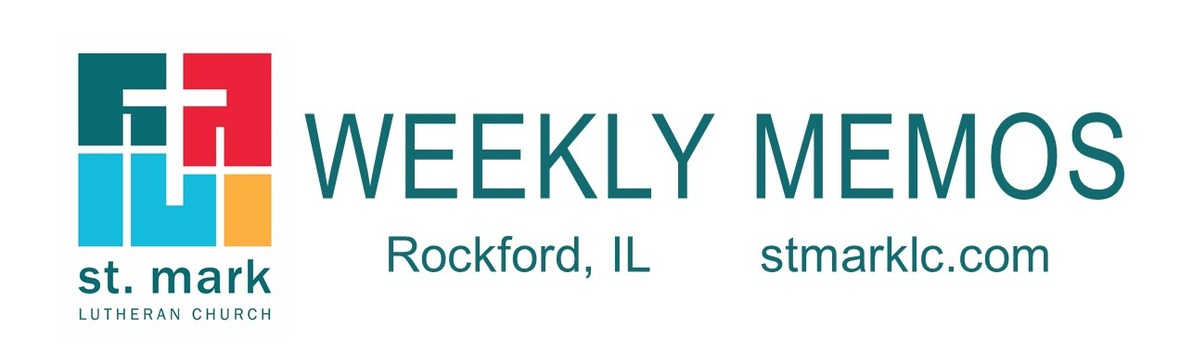table div table+table div table{width:100%;padding:0}table div table+table div table img{width:96.23%;padding:0;float:none}table div table+table div table td{width:100%;padding:0 1.88% 18px}/* styles */## Sunday, November 28 First Sunday of Advent

9:00 A.M. Worship with Live-Stream
10:00 A.M. Fellowship Hour with coffee and donuts
10:10 A.M. No Sunday School
10:10 A.M. Learning Hour/ Adult Forum
11:00 A.M. Worship in Fellowship Hall

## Tuesday, November 30

10:00 A.M. Staff Meeting
11:30 A.M. St. Mark Duplicate Bridge

## Wednesday, December 1

9:00 A.M. St. Mark Ringers
9:00 A.M. Women's Bible Study
9:00 A.M. Men's Bible Study
6:30 P.M. Confirmation
7:00 P.M. Voices of St. Mark

## Thursday, December 2

9:00 A.M. Cherry Valley Library Bridge Group
9:00 A.M. Primetimers Breakfast meeting at Stockholm Inn
6:00 P.M. Decorating St. Mark for Christmas
6:30 P.M. Emotions Anonymous
6:30 P.M. Blue Lights

## Friday, December 3

7:30 A.M. St. Mark Men's Breakfast Meeting at Denny's

## Saturday, December 4

10:00 A.M. Christmas Concert Rehearsal

## Sunday, December 5 Second Sunday of Advent

9:00 A.M. Worship with Live-Stream
10:00 A.M. Fellowship Hour with coffee and donuts
10:10 A.M. Sunday School
10:10 A.M. Learning Hour/ Adult Forum
11:00 A.M. Worship in Fellowship Hall
3:00 P.M. Christmas Concert

 table div table+table+table+table+table div table{width:100%;padding:0}table div table+table+table+table+table div table img{width:96.23%;padding:0;float:none}table div table+table+table+table+table div table td{width:100%;padding:0 1.88% 18px}/* styles */## News and Events

 table div table+table+table+table+table+table+table div table{width:100%;padding:0}table div table+table+table+table+table+table+table div table img{width:96.23%;padding:0;float:none}table div table+table+table+table+table+table+table div table td{width:100%;padding:0 1.88% 18px}/* styles */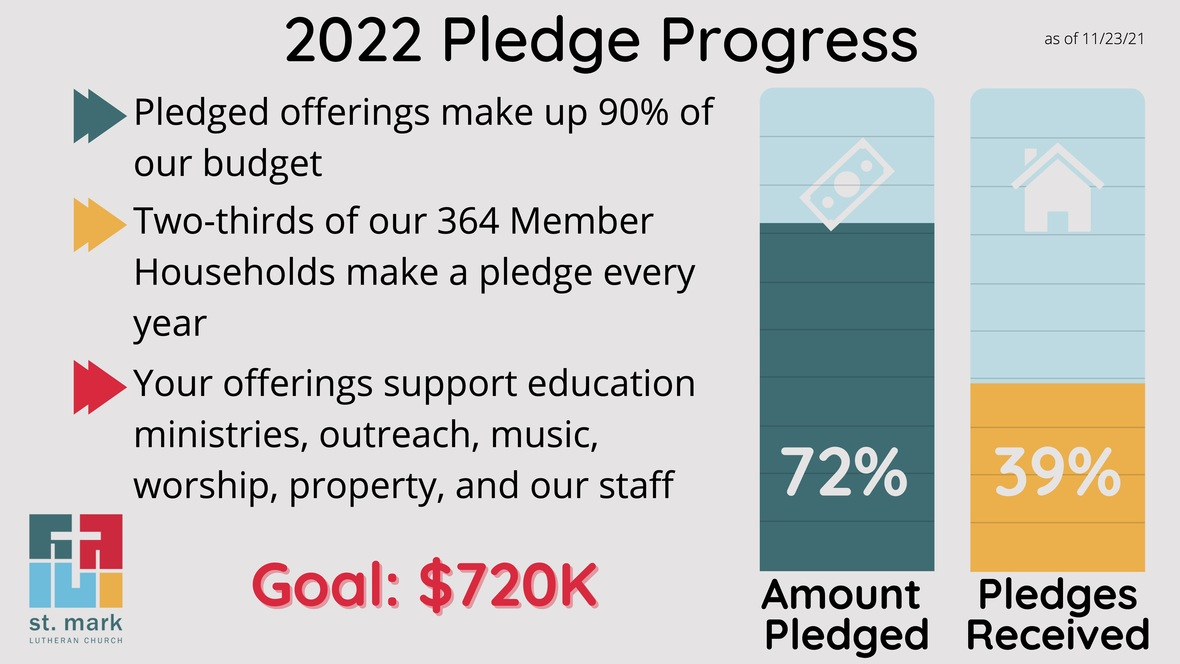/* styles */ Thank you to everyone who has submitted their pledge for 2022! We still have a long way to go to meet our goal. In order to plan for our 2022 ministries with confidence, we need everyone to respond. You can submit your plan for giving right now by following this link: 2022 Form, or we have pledge cards available on Sunday mornings or in the church office. Thank you for being part of the mission we share, by sharing your time, your gifts, and yourself with our wonderful community at St. Mark!
 table div table+table+table+table+table+table+table+table+table+table div table{width:100%;padding:0}table div table+table+table+table+table+table+table+table+table+table div table img{width:96.23%;padding:0;float:none}table div table+table+table+table+table+table+table+table+table+table div table td{width:100%;padding:0 1.88% 18px}/* styles */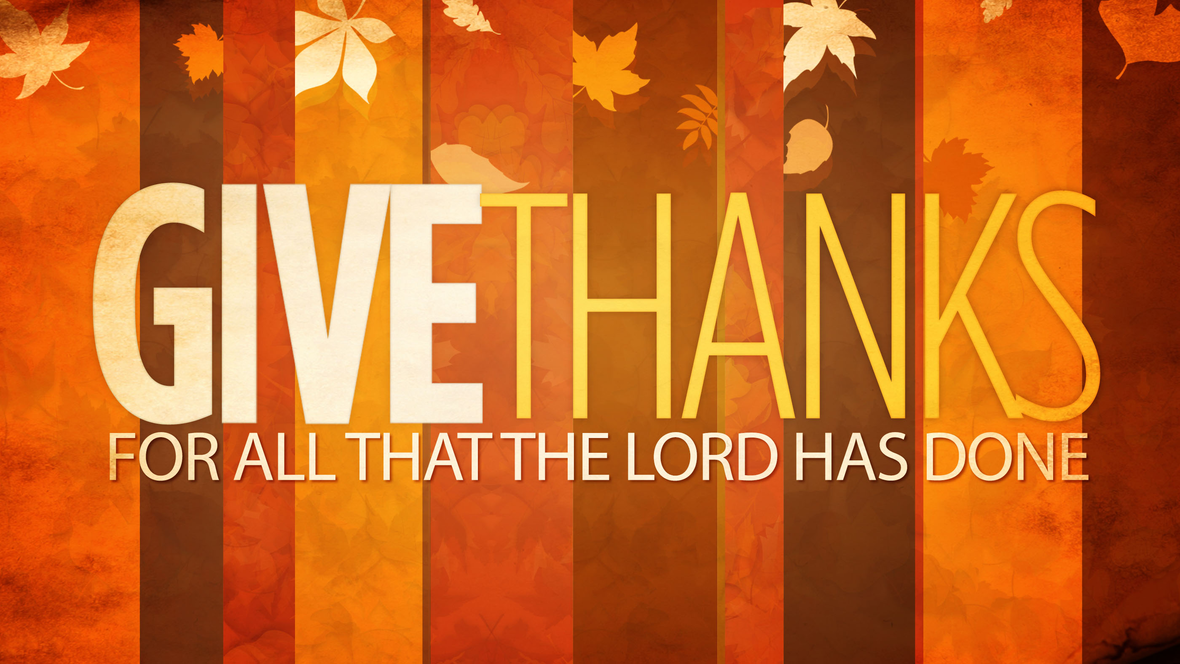Thanksgiving Eve Worship
Join us for worship on Wednesday, November 24, at 6:45 P.M. and enjoy fun, treats, and fellowship after the service in the cafe. If you are unable to join us tonight, click on this link at 6:45.

This year 100% of the offering will be donated to ELCA World Hunger. Also, you are encouraged to bring non-perishable food items to be donated to the Northern Illinois Food Bank. Give thanks this holiday season.

 table div table+table+table+table+table+table+table+table+table+table+table+table div table{width:100%;padding:0}table div table+table+table+table+table+table+table+table+table+table+table+table div table img{width:96.23%;padding:0;float:none}table div table+table+table+table+table+table+table+table+table+table+table+table div table td{width:100%;padding:0 1.88% 18px}/* styles *//* styles */ First Sunday of Advent 9:00 am - Traditional Worship in the Sanctuary (Prelude will begin at 8:55) 10:10 am - Sunday School, Adult Forum and Fellowship Hour with Coffee & Donuts 11:00 am - Blended Praise Service - Fellowship Hall Our weekly live-stream will occur during the 9:00 am Sanctuary service, with the prelude starting at 8:55. Follow this link for the live-stream service Sunday.
 table div table+table+table+table+table+table+table+table+table+table+table+table+table+table div table{width:100%;padding:0}table div table+table+table+table+table+table+table+table+table+table+table+table+table+table div table img{width:96.23%;padding:0;float:none}table div table+table+table+table+table+table+table+table+table+table+table+table+table+table div table td{width:100%;padding:0 1.88% 18px}/* styles *//* styles */ Sunday morning Adult Forum Our Sunday morning Adult Forum is during the learning hour at 10:10 am. Bring your coffee and a donut to the Adult Ministry room and enjoy the subject "My Soul Proclaims Your Greatness: a Closer look at the Psalms of Advent, led by Pastor Chad. There is no Sunday School this Sunday.
 table div table+table+table+table+table+table+table+table+table+table+table+table+table+table+table+table div table{width:100%;padding:0}table div table+table+table+table+table+table+table+table+table+table+table+table+table+table+table+table div table img{width:96.23%;padding:0;float:none}table div table+table+table+table+table+table+table+table+table+table+table+table+table+table+table+table div table td{width:100%;padding:0 1.88% 18px}/* styles */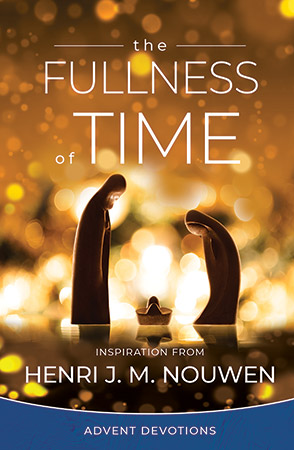Advent begins on Sunday, November 28. There are devotional booklets for you to pick up on the tables before you enter the Sanctuary or in the church office. Celebrate Advent and draw closer to God through the daily reflections and prayers inspired by Scripture and the spiritual writing of Henri J. M. Nouwen, The Fullness of Time.

 table div table+table+table+table+table+table+table+table+table+table+table+table+table+table+table+table+table+table div table{width:100%;padding:0}table div table+table+table+table+table+table+table+table+table+table+table+table+table+table+table+table+table+table div table img{width:96.23%;padding:0;float:none}table div table+table+table+table+table+table+table+table+table+table+table+table+table+table+table+table+table+table div table td{width:100%;padding:0 1.88% 18px}/* styles */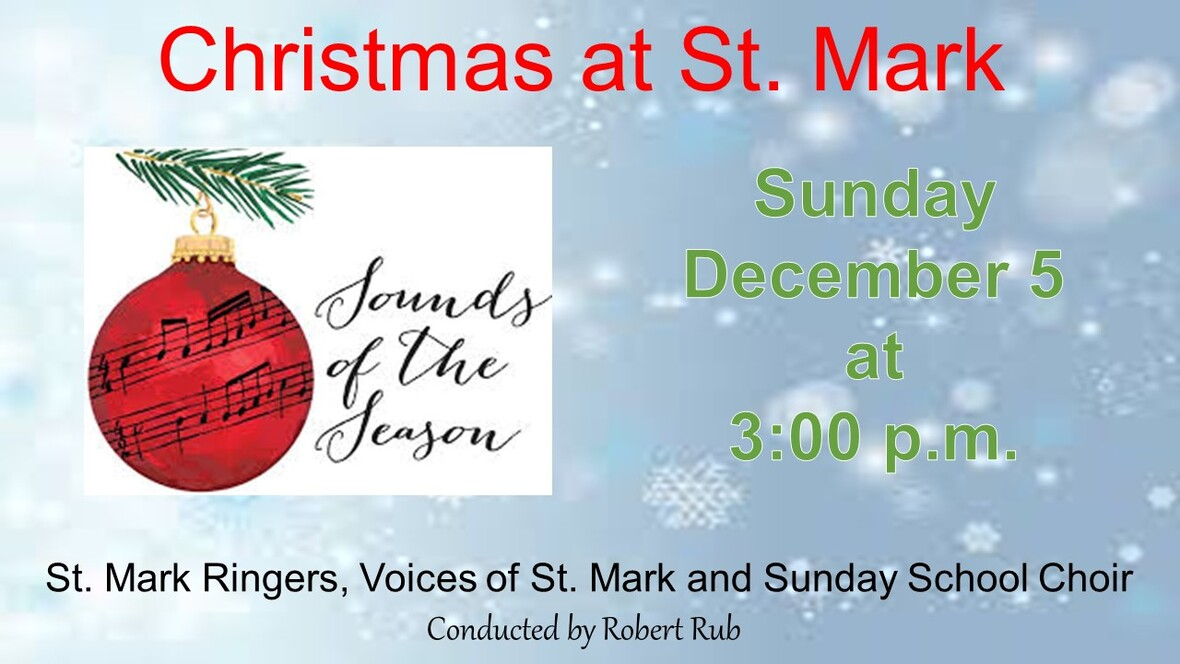table div table+table+table+table+table+table+table+table+table+table+table+table+table+table+table+table+table+table+table+table div table{width:100%;padding:0}table div table+table+table+table+table+table+table+table+table+table+table+table+table+table+table+table+table+table+table+table div table img{width:96.23%;padding:0;float:none}table div table+table+table+table+table+table+table+table+table+table+table+table+table+table+table+table+table+table+table+table div table td{width:100%;padding:0 1.88% 18px}/* styles */2022 Altar Flower Chart is Up

Each Sunday we have two beautiful bouquets of flowers on the altar. The 2022 flower chart is up in the narthex on the bulletin board. You may sign up on the chart, call the church office or click on this link. We will continue to purchase them from Cherry Blossom. The price per arrangement will be \$40, a slight increase from the past few years due to higher fresh flower costs. If you sign up online, put your memory or honor of in the comment line and pay here or write a check to St. Mark. If you have the mobile app you can do all of it on there. You may take your flowers home after the service or stop by the office during the week to pick them up.

 table div table+table+table+table+table+table+table+table+table+table+table+table+table+table+table+table+table+table+table+table+table+table div table{width:100%;padding:0}table div table+table+table+table+table+table+table+table+table+table+table+table+table+table+table+table+table+table+table+table+table+table div table img{width:96.23%;padding:0;float:none}table div table+table+table+table+table+table+table+table+table+table+table+table+table+table+table+table+table+table+table+table+table+table div table td{width:100%;padding:0 1.88% 18px}/* styles */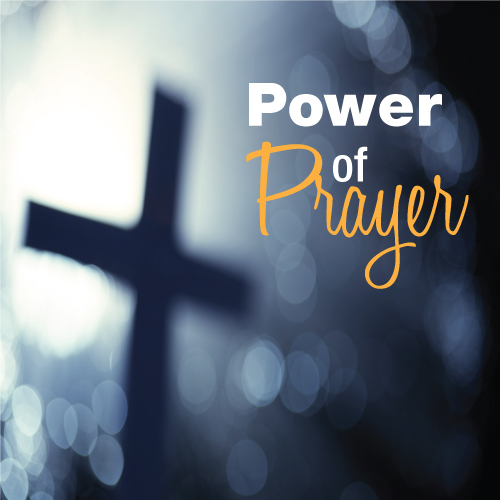St. Mark Prayer Chain
Would you like to be a part of the St. Mark prayer chain? Prayer requests come into the church office weekly. As we receive prayer requests we would send out an e-mail and ask that you pray daily for the persons mentioned in the requests. All requests are to remain confidential. Please e-mail melinda@stmarklc.com if you would like to participate in the prayer chain. “Be anxious for nothing, but in everything by prayer and supplication, with thanksgiving, let your requests be made known to God.” Colossians 4:2.

 table div table+table+table+table+table+table+table+table+table+table+table+table+table+table+table+table+table+table+table+table+table+table+table+table div table{width:100%;padding:0}table div table+table+table+table+table+table+table+table+table+table+table+table+table+table+table+table+table+table+table+table+table+table+table+table div table img{width:96.23%;padding:0;float:none}table div table+table+table+table+table+table+table+table+table+table+table+table+table+table+table+table+table+table+table+table+table+table+table+table div table td{width:100%;padding:0 1.88% 18px}/* styles *//* styles */ St. Mark was able to help McIntosh School with Turkey Bingo, forty turkey’s and pies! Thank you to the Outreach committee, our pastors, and the volunteers that helped to make this happen.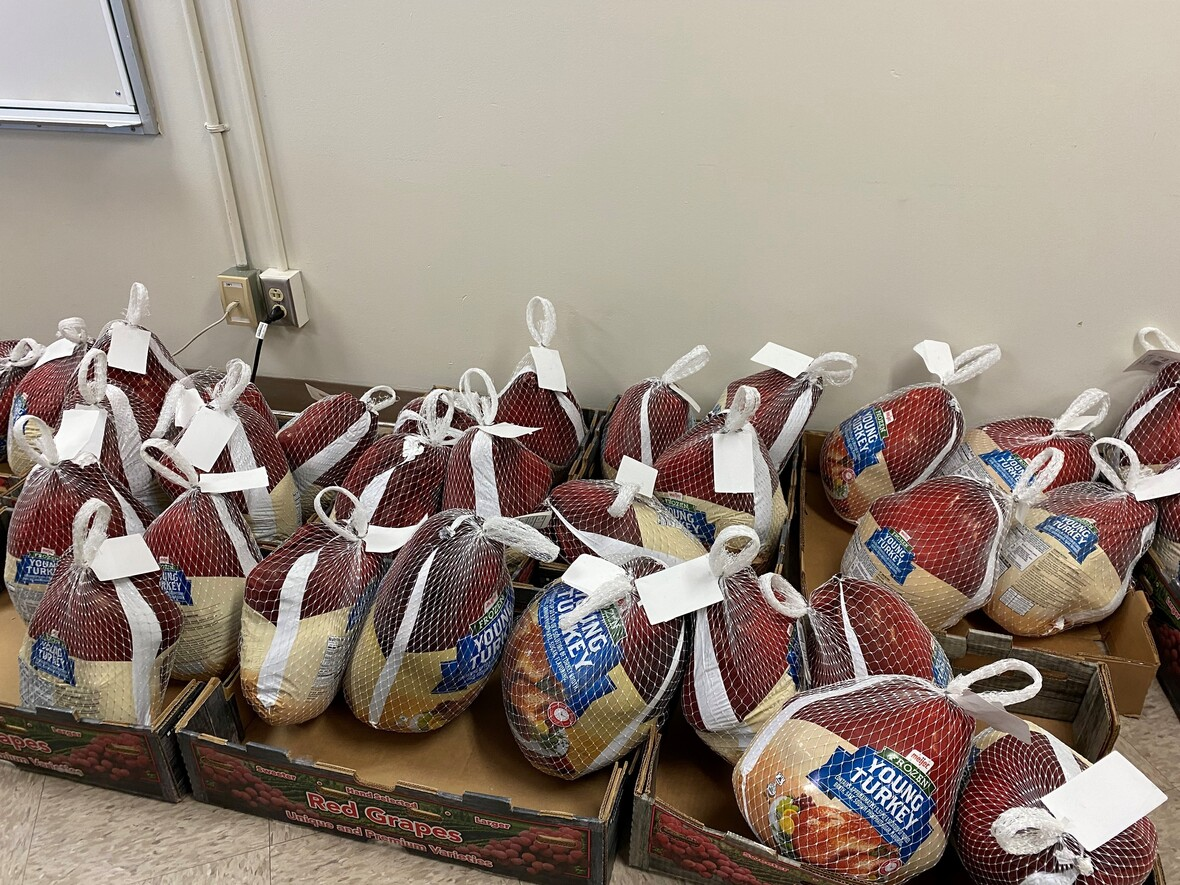table div table+table+table+table+table+table+table+table+table+table+table+table+table+table+table+table+table+table+table+table+table+table+table+table+table+table+table div table{width:100%;padding:0}table div table+table+table+table+table+table+table+table+table+table+table+table+table+table+table+table+table+table+table+table+table+table+table+table+table+table+table div table img{width:96.23%;padding:0;float:none}table div table+table+table+table+table+table+table+table+table+table+table+table+table+table+table+table+table+table+table+table+table+table+table+table+table+table+table div table td{width:100%;padding:0 1.88% 18px}/* styles */Wassail Sunday

Please drop off your cookies in a decorative tin at the church kitchen by 9:00 am on December 12. Remember to label your tin with your name so we can return it to you.

 table div table+table+table+table+table+table+table+table+table+table+table+table+table+table+table+table+table+table+table+table+table+table+table+table+table+table+table+table+table div table{width:100%;padding:0}table div table+table+table+table+table+table+table+table+table+table+table+table+table+table+table+table+table+table+table+table+table+table+table+table+table+table+table+table+table div table img{width:96.23%;padding:0;float:none}table div table+table+table+table+table+table+table+table+table+table+table+table+table+table+table+table+table+table+table+table+table+table+table+table+table+table+table+table+table div table td{width:100%;padding:0 1.88% 18px}/* styles */The holiday season is just around the corner and your shopping can help make a difference, at no additional cost to you!
When you shop at smile.amazon.com or in the Amazon app with AmazonSmile turned on within settings, you'll find the same products and same low prices as the Amazon you already know-plus, they donate a portion of your purchases to us, St. Mark.

You can select St. Mark as your AmazonSmile charity by visiting: AmazonSmile link

 table div table+table+table+table+table+table+table+table+table+table+table+table+table+table+table+table+table+table+table+table+table+table+table+table+table+table+table+table+table+table+table div table{width:100%;padding:0}table div table+table+table+table+table+table+table+table+table+table+table+table+table+table+table+table+table+table+table+table+table+table+table+table+table+table+table+table+table+table+table div table img{width:96.23%;padding:0;float:none}table div table+table+table+table+table+table+table+table+table+table+table+table+table+table+table+table+table+table+table+table+table+table+table+table+table+table+table+table+table+table+table div table td{width:100%;padding:0 1.88% 18px}/* styles */Come help Rosie’s Birthday Club assemble “gingerbread” kits.
St. Mark is gathering some volunteers to help. There are three shifts available December 9th, or 10th 4:30-8:30 P.M. and December 11, 7:00-9:00 A.M. at Guildford High School. Please sign-up on Sunday morning in Fellowship Hall or e-mail melinda@stmarklc.com

 table div table+table+table+table+table+table+table+table+table+table+table+table+table+table+table+table+table+table+table+table+table+table+table+table+table+table+table+table+table+table+table+table+table div table{width:100%;padding:0}table div table+table+table+table+table+table+table+table+table+table+table+table+table+table+table+table+table+table+table+table+table+table+table+table+table+table+table+table+table+table+table+table+table div table img{width:96.23%;padding:0;float:none}table div table+table+table+table+table+table+table+table+table+table+table+table+table+table+table+table+table+table+table+table+table+table+table+table+table+table+table+table+table+table+table+table+table div table td{width:100%;padding:0 1.88% 18px}/* styles *//* styles */ Place your order for Swedish Korv! See below.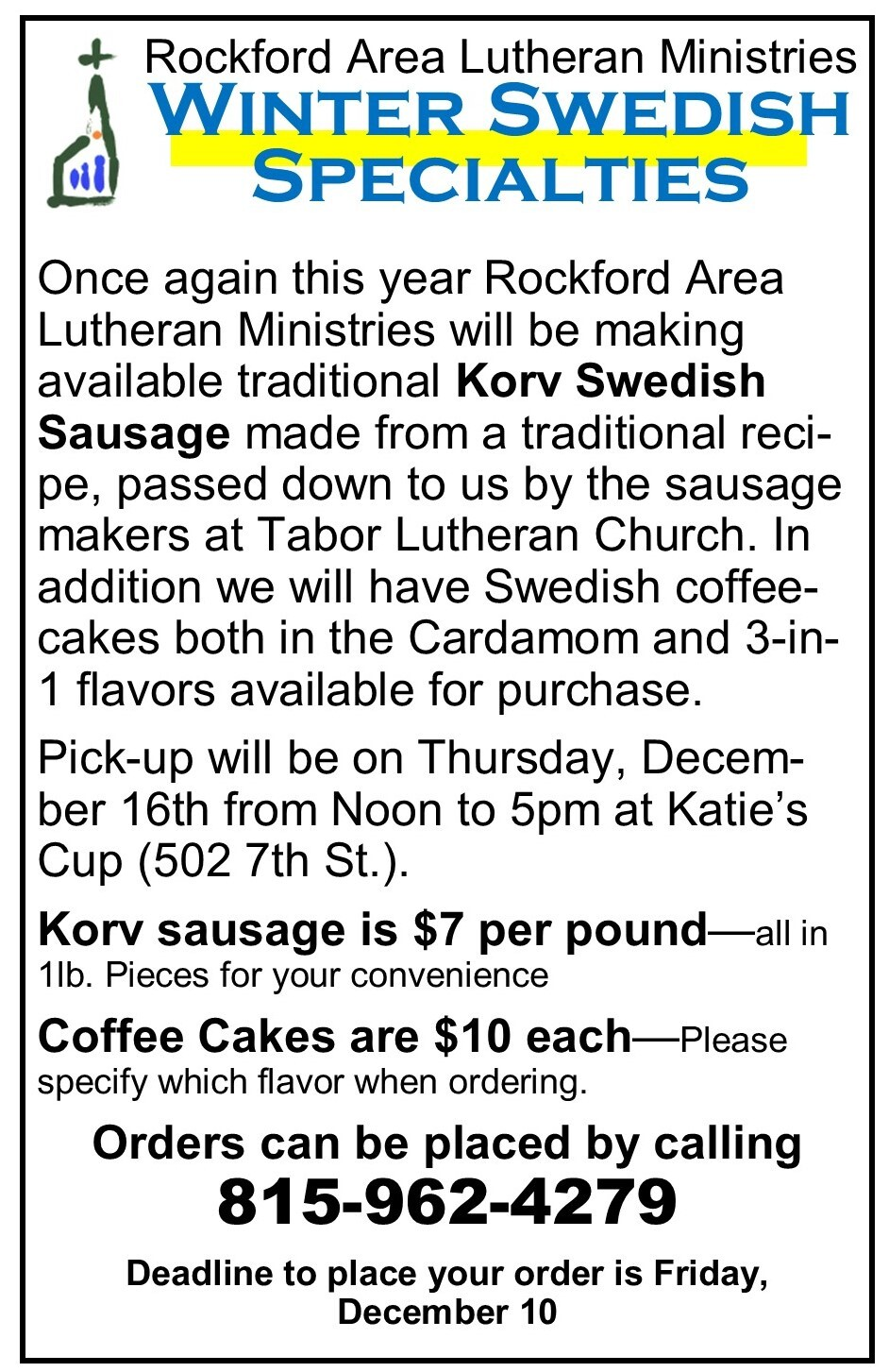table div table+table+table+table+table+table+table+table+table+table+table+table+table+table+table+table+table+table+table+table+table+table+table+table+table+table+table+table+table+table+table+table+table+table+table+table div table{width:100%;padding:0}table div table+table+table+table+table+table+table+table+table+table+table+table+table+table+table+table+table+table+table+table+table+table+table+table+table+table+table+table+table+table+table+table+table+table+table+table div table img{width:96.23%;padding:0;float:none}table div table+table+table+table+table+table+table+table+table+table+table+table+table+table+table+table+table+table+table+table+table+table+table+table+table+table+table+table+table+table+table+table+table+table+table+table div table td{width:100%;padding:0 1.88% 18px}/* styles */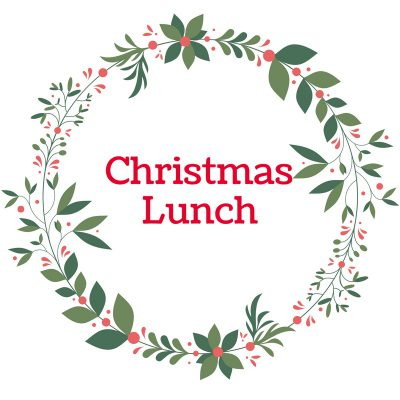In December Miriam Circle will meet for a Christmas Luncheon.
Time: Wednesday, December 8 at 12 Noon
Place: Tavern on Clark

All women are welcome to join us for this time of fellowship.
Please RSVP to Betty Sparks: Phone 815 636-1122, Email kbsp4968@msn.com

 table div table+table+table+table+table+table+table+table+table+table+table+table+table+table+table+table+table+table+table+table+table+table+table+table+table+table+table+table+table+table+table+table+table+table+table+table+table+table div table{width:100%;padding:0}table div table+table+table+table+table+table+table+table+table+table+table+table+table+table+table+table+table+table+table+table+table+table+table+table+table+table+table+table+table+table+table+table+table+table+table+table+table+table div table img{width:96.23%;padding:0;float:none}table div table+table+table+table+table+table+table+table+table+table+table+table+table+table+table+table+table+table+table+table+table+table+table+table+table+table+table+table+table+table+table+table+table+table+table+table+table+table div table td{width:100%;padding:0 1.88% 18px}/* styles */Festival of Lights!
32 Years of Lighting up the holidays! For the first time, St. Mark has a display and is participating in the festival (Sinnissippi Park 1401 N. Second St.) You’ll want to drive-thru and check it out! The lights will be lit starting November 26th-28th from 5-11P.M. December 2-19th Thursday through Sunday. Extended hours December 20-31. www.4thandLights.com

 table div table+table+table+table+table+table+table+table+table+table+table+table+table+table+table+table+table+table+table+table+table+table+table+table+table+table+table+table+table+table+table+table+table+table+table+table+table+table+table+table div table{width:100%;padding:0}table div table+table+table+table+table+table+table+table+table+table+table+table+table+table+table+table+table+table+table+table+table+table+table+table+table+table+table+table+table+table+table+table+table+table+table+table+table+table+table+table div table img{width:96.23%;padding:0;float:none}table div table+table+table+table+table+table+table+table+table+table+table+table+table+table+table+table+table+table+table+table+table+table+table+table+table+table+table+table+table+table+table+table+table+table+table+table+table+table+table+table div table td{width:100%;padding:0 1.88% 18px}/* styles */## Youth Ministry

 table div table+table+table+table+table+table+table+table+table+table+table+table+table+table+table+table+table+table+table+table+table+table+table+table+table+table+table+table+table+table+table+table+table+table+table+table+table+table+table+table+table+table div table{width:100%;padding:0}table div table+table+table+table+table+table+table+table+table+table+table+table+table+table+table+table+table+table+table+table+table+table+table+table+table+table+table+table+table+table+table+table+table+table+table+table+table+table+table+table+table+table div table img{width:96.23%;padding:0;float:none}table div table+table+table+table+table+table+table+table+table+table+table+table+table+table+table+table+table+table+table+table+table+table+table+table+table+table+table+table+table+table+table+table+table+table+table+table+table+table+table+table+table+table div table td{width:100%;padding:0 1.88% 18px}/* styles */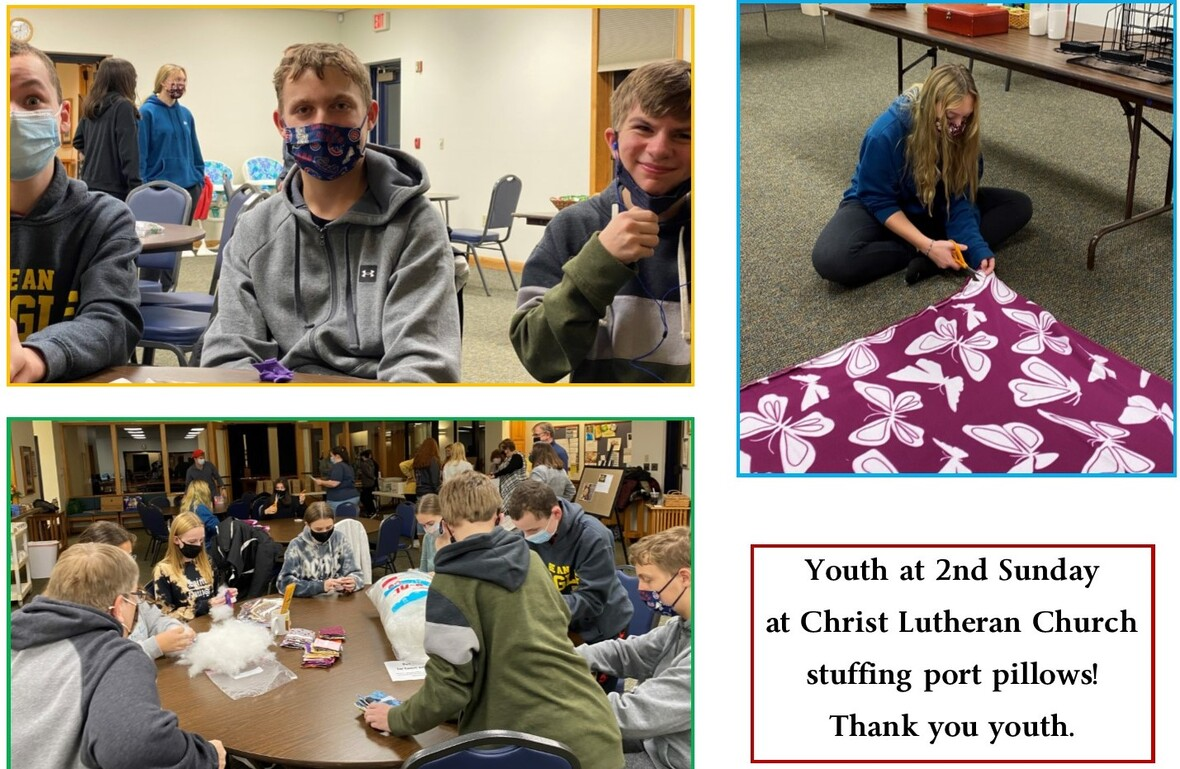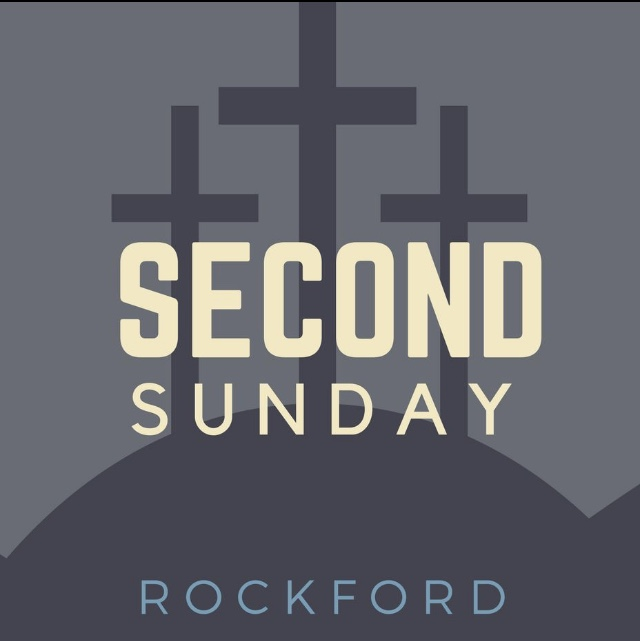Second Sunday met at Christ Lutheran last Sunday. We assembled CARE packages and filled “port” pillows. The next Second Sunday we will have a Christmas Party at Grace Lutheran in Loves Park.

 table div table+table+table+table+table+table+table+table+table+table+table+table+table+table+table+table+table+table+table+table+table+table+table+table+table+table+table+table+table+table+table+table+table+table+table+table+table+table+table+table+table+table+table+table+table div table{width:100%;padding:0}table div table+table+table+table+table+table+table+table+table+table+table+table+table+table+table+table+table+table+table+table+table+table+table+table+table+table+table+table+table+table+table+table+table+table+table+table+table+table+table+table+table+table+table+table+table div table img{width:96.23%;padding:0;float:none}table div table+table+table+table+table+table+table+table+table+table+table+table+table+table+table+table+table+table+table+table+table+table+table+table+table+table+table+table+table+table+table+table+table+table+table+table+table+table+table+table+table+table+table+table+table div table td{width:100%;padding:0 1.88% 18px}/* styles */## Outreach Ministry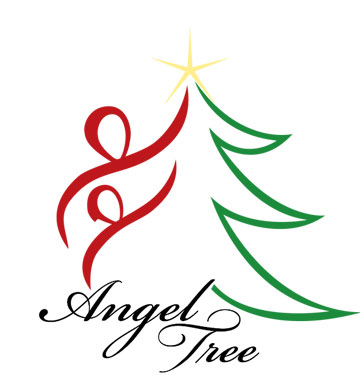Wrapped "Angel" Gifts Due back by Sunday, November 28.
Thank you to all that have taken home an "Angel" tag for LSSI, Lutheran Social Services. Your wrapped gift should be returned to St. Mark by Sunday, November 28. Thank you for making a Foster child's Christmas a special one.

 table div table+table+table+table+table+table+table+table+table+table+table+table+table+table+table+table+table+table+table+table+table+table+table+table+table+table+table+table+table+table+table+table+table+table+table+table+table+table+table+table+table+table+table+table+table+table+table+table div table{width:100%;padding:0}table div table+table+table+table+table+table+table+table+table+table+table+table+table+table+table+table+table+table+table+table+table+table+table+table+table+table+table+table+table+table+table+table+table+table+table+table+table+table+table+table+table+table+table+table+table+table+table+table div table img{width:96.23%;padding:0;float:none}table div table+table+table+table+table+table+table+table+table+table+table+table+table+table+table+table+table+table+table+table+table+table+table+table+table+table+table+table+table+table+table+table+table+table+table+table+table+table+table+table+table+table+table+table+table+table+table+table div table td{width:100%;padding:0 1.88% 18px}/* styles */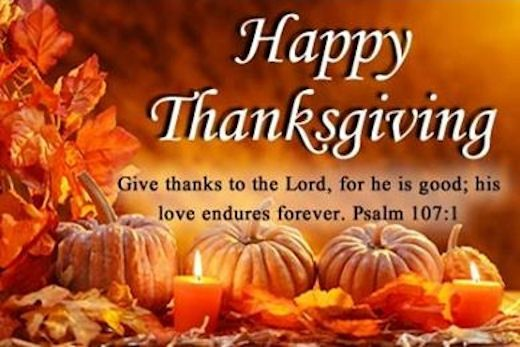table div table+table+table+table+table+table+table+table+table+table+table+table+table+table+table+table+table+table+table+table+table+table+table+table+table+table+table+table+table+table+table+table+table+table+table+table+table+table+table+table+table+table+table+table+table+table+table+table+table+table div table{width:100%;padding:0}table div table+table+table+table+table+table+table+table+table+table+table+table+table+table+table+table+table+table+table+table+table+table+table+table+table+table+table+table+table+table+table+table+table+table+table+table+table+table+table+table+table+table+table+table+table+table+table+table+table+table div table img{width:96.23%;padding:0;float:none}table div table+table+table+table+table+table+table+table+table+table+table+table+table+table+table+table+table+table+table+table+table+table+table+table+table+table+table+table+table+table+table+table+table+table+table+table+table+table+table+table+table+table+table+table+table+table+table+table+table+table div table td{width:100%;padding:0 1.88% 18px}/* styles */## Our St. Mark members:

John Johnson Gary York, Russ Oellig, Tim Vivian, John Lund, Dennis Clark, Linda Vivian, Jack Sowl, Lorine Kortus, Jan Cotter, Diane Swanson, Sherri Lindquist, Bette Patterson, Keith Haggestad.

## Family and friends of St. Mark members:

Izzy Olson, Arthur Graham, Rick Norser, Jenny Hobby, Bill King, Jeanne Menzies, Chris Nack, Katelynn Audette, Mike Glynn, Shirley Brown, Ron Ganschow, Bill Ganschow, Clyde Webster, Bernice, Conrad Semmelroth, Nici King, Debbie Adams, Linda Johnson, Eva Love Sherbondy, Norma Comstock, Carolyn King.

## Those serving in the military and their families:

Hunter Haggestad, Tristan Davenport, Scot Hornick,
Bailey Panjkovich, Jim Tammen, Brandon Ballenger, Taylor Eversole, Dean Barron.

Strengthen and encourage those in public health services and in the medical profession: care-givers, nurses, attendants, doctors, all who commit themselves to care for the sick and their families.

 table div table+table+table+table+table+table+table+table+table+table+table+table+table+table+table+table+table+table+table+table+table+table+table+table+table+table+table+table+table+table+table+table+table+table+table+table+table+table+table+table+table+table+table+table+table+table+table+table+table+table+table+table+table div table{width:100%;padding:0}table div table+table+table+table+table+table+table+table+table+table+table+table+table+table+table+table+table+table+table+table+table+table+table+table+table+table+table+table+table+table+table+table+table+table+table+table+table+table+table+table+table+table+table+table+table+table+table+table+table+table+table+table+table div table img{width:96.23%;padding:0;float:none}table div table+table+table+table+table+table+table+table+table+table+table+table+table+table+table+table+table+table+table+table+table+table+table+table+table+table+table+table+table+table+table+table+table+table+table+table+table+table+table+table+table+table+table+table+table+table+table+table+table+table+table+table+table div table td{width:100%;padding:0 1.88% 18px}/* styles *//* styles */ Office Hours Monday through Friday 9:00 A.M. - 4:00 P.M. Telephone: 815-398-3557 Publications St. Mark Memos, the weekly e-newsletter, is distributed each Thursday. The deadline for submission is Monday by 3:00 P.M. Please click to send submissions. Worship Services Miss a Sunday at St. Mark? Please visit our website and watch the service online. Please click to watch.
 table div table+table+table+table+table+table+table+table+table+table+table+table+table+table+table+table+table+table+table+table+table+table+table+table+table+table+table+table+table+table+table+table+table+table+table+table+table+table+table+table+table+table+table+table+table+table+table+table+table+table+table+table+table+table+table div table{width:100%;padding:0}table div table+table+table+table+table+table+table+table+table+table+table+table+table+table+table+table+table+table+table+table+table+table+table+table+table+table+table+table+table+table+table+table+table+table+table+table+table+table+table+table+table+table+table+table+table+table+table+table+table+table+table+table+table+table+table div table img{width:96.23%;padding:0;float:none}table div table+table+table+table+table+table+table+table+table+table+table+table+table+table+table+table+table+table+table+table+table+table+table+table+table+table+table+table+table+table+table+table+table+table+table+table+table+table+table+table+table+table+table+table+table+table+table+table+table+table+table+table+table+table+table div table td{width:100%;padding:0 1.88% 18px}/* styles */